# 0.3: Functions

$$\newcommand{\vecs}{\overset { \rightharpoonup} {\mathbf{#1}} }$$ $$\newcommand{\vecd}{\overset{-\!-\!\rightharpoonup}{\vphantom{a}\smash {#1}}}$$$$\newcommand{\id}{\mathrm{id}}$$ $$\newcommand{\Span}{\mathrm{span}}$$ $$\newcommand{\kernel}{\mathrm{null}\,}$$ $$\newcommand{\range}{\mathrm{range}\,}$$ $$\newcommand{\RealPart}{\mathrm{Re}}$$ $$\newcommand{\ImaginaryPart}{\mathrm{Im}}$$ $$\newcommand{\Argument}{\mathrm{Arg}}$$ $$\newcommand{\norm}{\| #1 \|}$$ $$\newcommand{\inner}{\langle #1, #2 \rangle}$$ $$\newcommand{\Span}{\mathrm{span}}$$ $$\newcommand{\id}{\mathrm{id}}$$ $$\newcommand{\Span}{\mathrm{span}}$$ $$\newcommand{\kernel}{\mathrm{null}\,}$$ $$\newcommand{\range}{\mathrm{range}\,}$$ $$\newcommand{\RealPart}{\mathrm{Re}}$$ $$\newcommand{\ImaginaryPart}{\mathrm{Im}}$$ $$\newcommand{\Argument}{\mathrm{Arg}}$$ $$\newcommand{\norm}{\| #1 \|}$$ $$\newcommand{\inner}{\langle #1, #2 \rangle}$$ $$\newcommand{\Span}{\mathrm{span}}$$$$\newcommand{\AA}{\unicode[.8,0]{x212B}}$$

### Functions

Mapping

Let $$A$$ be a set.

Then $$A \times A$$ is the Cartesian product of $$A$$.

Example:  If $$A = \mathbb{R}$$ then this is the Cartesian plane -  Like $$\{x,y: x,y \in A\}$$.

A function $$f$$ is a subset (symbol is $$\subseteq$$) of $$A \times B$$ such that for every $$a \in A$$ there exists a unique $$b \in B$$ such that $$f(a)=b$$.Denoted by $$f: A \rightarrow B$$ or $$A \xrightarrow{f} B$$.

$$A$$ is the domain of the function $$f$$.

$$B$$ is the co-domain of the function $$f$$.

The range of $$f$$ is $$f(A)=\big{\{}f(x): x \in A \big{\}} \subseteq B$$

A well-defined function is one that for every value of $$A$$, there is one and only one value of $$B$$.

Definition:

Let $$f$$ be a function from $$A \rightarrow B$$.

1. $$f$$ is called onto (surjective) if $$f(A)=B$$.

2. $$f$$ is called one to one (injective) if $$f(x_1)=f(x_2), x_1,x_2 \in A$$ then $$x_1=x_2$$.

3. $$f$$ is bijective if it is surjective and injective.

4. A function can be injective, surjective, bijective (both injective & surjective), or neither.

Note:

Let $$f: A \rightarrow B$$.  If $$f$$ is 1-1 and onto then $$f$$ is a bijection from $$A \rightarrow B.$$

If there is a bijection between two finite sets, the number of elements in each set is the same.  Notationally:  |A| = |B|.

##### Example $$\PageIndex{1}$$##### Example $$\PageIndex{2}$$

Consider the mapping:Is this mapping

a) Well defined?

b)  Is it surjective?

c) Is it injective?

d) Is it bijective?

###### Solution

The function is well-defined. Not injective. Not subjective, not bijective.

##### Example $$\PageIndex{3}$$

Consider the mapping:Is this mapping

a) Well defined?

b)  Is it surjective?

c) Is it injective?

d) Is it bijective?

###### Solution

This is well defined bijective function.

##### Example $$\PageIndex{4}$$

Given $$f: \mathbb{Q} \rightarrow \mathbb{Z}$$ where $$\mathbb{Q}=\{\frac{a}{b}:a,b \in \mathbb{Z}\}$$ and $$f(\frac{a}{b})=a$$.  Is this a well-defined function?

###### Solution

Let $$A$$ and $$B$$ be sets such that $$A=\{\frac{a}{b}:a,b \in \mathbb{Z}\}$$ and $$B=\mathbb{Z}$$.

Using a counterexample, $$f(\frac{a}{b})$$ is not a well-defined function since $$f(\frac{1}{2})=1$$ and $$f(\frac{2}{4})=2$$, but $$\frac{1}{2}=\frac{2}{4}$$.  This is an example of an item in $$A$$ that maps to more than one element in $$B$$.

Example $$\PageIndex{5}$$

Let

$$\mathbb{Q}=\big{\{}\frac{a}{b}: b\ne 0,\text{and } gcd(a,b)=1 \big{\}}$$

and $$f: \mathbb{Q} \rightarrow \mathbb{Z}$$ that is defined by

$$f(\frac{a}{b})=a.$$

a) Is $$f$$ a well-defined function? b)  Is it surjective?  c) Is it injective?  d) Is it bijective?

Solution

1. The function is well-defined.

Let $$A$$ and $$B$$ be set.

Let $$a \in A$$.

We need to show that if $$f(a)=b$$ then $$b\in B$$ and if $$f(a)=b_1$$ that $$b=b_1$$.

We are given $$f: \mathbb{Q} \rightarrow \mathbb{Z}$$, which means that this is a function thus $$f(a)$$ will map to one and only one element of $$B$$.  Thus if $$f(a)=b$$ and if $$f(a)=b_1$$ then $$b=b_1$$.

In b) below, we have shown that the function is surjective, thus $$f(a)=b$$,

1. The function is surjective

Proof:

We shall show that $$f(\mathbb{Q})=\mathbb{Z}$$ by showing that  $$f(\mathbb{Q}) \subseteq \mathbb{Z}$$ and  $$\mathbb{Z} \subseteq f(\mathbb{Q})$$.

Since we are given $$f: \mathbb{Q} \rightarrow \mathbb{Z}$$,  $$f(\mathbb{Q}) \subseteq \mathbb{Z}$$.

Next, we shall show that $$\mathbb{Z} \subseteq f(\mathbb{Q})$$.

Let $$n \in \mathbb{Z}$$, then $$\frac{n}{1} \in \mathbb{Q}$$ and $$f\big(\frac{n}{1}\big)=n$$.

Hence $$n \in f(\mathbb{Q})$$.

Hence $$\mathbb{Z} \subseteq f(\mathbb{Q})$$.

Since $$\mathbb{Z} \subseteq f(\mathbb{Q})$$ and $$f(\mathbb{Q}) \subseteq \mathbb{Z}, f(\mathbb{Q})=\mathbb{Z}$$.◻

1. The function is not injective.

Counterexample:

Let $$A=\big{\{}\frac{a}{b}: b\ne 0,\text{and } gcd(a,b)=1 \big{\}}$$ and $$B=\mathbb{Z}$$.

Note $$\frac{1}{4}, \frac{1}{3} \in A$$ and that $$f(\frac{1}{4}), f(\frac{1}{3}) \in B$$ and that $$f(\frac{1}{4})=f(\frac{1}{3})=1$$.

Since $$\frac{1}{4} \ne \frac{1}{3}$$ , the function is not injective.

1. The function is not bijective since it has been shown not to be both injective and surjective.

## Operation on functions: Composition

##### Definition:Composition of functions

Let $$f: A \rightarrow B$$ and $$g: B \rightarrow C$$. Then the composition functions of $$g$$ and $$f$$, $$g \circ f: A \to C$$, defined by

$$(g \circ f)(a)=g(f(a)), \; \forall a \in A$$.##### Definition: Identity function

Identity function $$id_A: A \rightarrow A$$ defined by $$id_A(x)=x, \forall x \in A$$.

##### Definition: Inverse of a function

Let $$f: A \rightarrow B$$ then $$f$$ has an inverse $$g: B \rightarrow A$$ if $$(f \circ g)= id_B$$ and $$(g \circ f)=id_A$$.  Note the domain of $$f \circ g$$ is the domain of $$g$$.

Let $$f:A \rightarrow B$$ be a bijection.  Then the inverse of $$f$$, denoted by $$f^{-1}:B \rightarrow A$$ defined by $$f^{-1}(b)=a$$ if $$f(a)=b$$ if $$a \in A$$ and $$b \in B$$.

Note: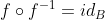and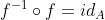.##### Example $$\PageIndex{6}$$

Let $$f: A \rightarrow B$$ and $$g: B \rightarrow C$$. Then.

1. If $$f$$ and $$g$$ are surjective, then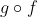is surjective.

Proof:

We will show thatis surjective.

Let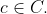Since g is surjective, there exists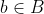such thatSince f is surjective, there exists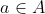such that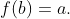Hence there existssuch that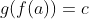.

Therefore, if $$f$$ and $$g$$ are surjective, thenis surjective.◻

(better proof) If $$f$$ is onto and $$g$$ is onto then $$g \circ f$$ is onto.

Proof:

We shall show that $$(g \circ f)(A)=C$$.

Since $$f$$ and $$g$$ are onto, by definition of a function, $$(g \circ f)(A) \subseteq C$$.

Now we need to show that $$C \subseteq (g \circ f)(A)$$.

Let $$c \in C$$.

Since $$g$$ is onto $$\exists b \in B$$ s.t. $$c=g(b)$$.

Since $$f$$ is onto, $$\exists a \in A$$ s.t. $$b=f(a)$$.

Consider $$(g \circ f)(a)=g(f(a))$$ (by definition)

$$=g(b)$$

$$=c$$.

Therefore $$c \in (g \circ f)(A)$$.

Hence $$C \subseteq (g \circ f)(A)$$.

Since $$(g \circ f)(A) \subseteq C$$ and $$C \subseteq (g \circ f)(A)$$, then $$g \circ f$$ is onto.◻

##### Example $$\PageIndex{7}$$
1. If $$f$$ and $$g$$ are injective, thenis injective. That is,

If $$f$$ is 1-1 and $$g$$ is 1-1 then $$g \circ f$$ is 1-1.

Proof:

Letsuch that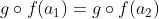.

Then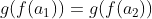.

Since  $$g$$ is 1-1,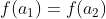.

Since,  $$f$$ is 1-1,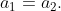Henceis injective.

##### Example $$\PageIndex{8}$$

Given $$A$$ is a matrix and $$T_A:\mathbb{R}^2 \rightarrow \mathbb{R}^2$$ defined by $$T_A \begin{bmatrix} x\\ y\end{bmatrix} = \begin{bmatrix} 1 &1\\ 0 & 1\end{bmatrix} \begin{bmatrix} x\\ y\end{bmatrix}$$.  Note this is a matrix transformation which is linear.

1. Is $$T_A$$ 1-1?

Yes, $$T_A$$ is 1-1.

Proof:

Let $$\begin{bmatrix} x_1\\ y_1\end{bmatrix}$$and $$\begin{bmatrix} x_2\\ y_2\end{bmatrix} \in \mathbb{R}^2$$ s.t. $$T_A \begin{bmatrix} x_1\\ y_1\end{bmatrix} =T_A \begin{bmatrix} x_2\\ y_2\end{bmatrix}$$.

We will show that $$\begin{bmatrix} x_1\\ y_1\end{bmatrix} =\begin{bmatrix} x_2\\ y_2\end{bmatrix}$$.

$$T_A \begin{bmatrix} x\\ y\end{bmatrix} =\begin{bmatrix} x+y\\ y\end{bmatrix}$$.

Thus  $$\begin{bmatrix} x_1 +y_1\\ y_1\end{bmatrix} =\begin{bmatrix} x_2+y_2\\ y_2\end{bmatrix}$$.

Thus $$x_1+y_1=x_2+y_2$$ and $$y_1=y_2$$.

Thus $$x_1=x_2$$ and $$y_1=y_2$$.

Hence $$\begin{bmatrix} x_1\\ y_1\end{bmatrix} =\begin{bmatrix} x_2\\ y_2\end{bmatrix}$$.

Thus $$T_A$$ is 1-1.◻

1. Is $$T_A \begin{bmatrix} x\\ y\end{bmatrix}$$ onto?

Yes, $$T_A \begin{bmatrix} x\\ y\end{bmatrix}$$ is onto.

Proof:

We know by definition that $$T_A \begin{bmatrix} x\\ y\end{bmatrix} \subseteq \mathbb{R}^2$$.

We need to show that $$\mathbb{R}^2 \subseteq T_A \begin{bmatrix} x\\ y\end{bmatrix}$$.

Let $$\begin{bmatrix} a\\ b\end{bmatrix} \in \mathbb{R}^2$$.

Find $$\begin{bmatrix} x\\ y\end{bmatrix} \in \mathbb{R}^2$$ s.t. $$\begin{bmatrix} 1 &1\\ 0 & 1\end{bmatrix} \begin{bmatrix} x\\ y\end{bmatrix}=\begin{bmatrix} a\\ b\end{bmatrix}$$.

$$\begin{bmatrix} x+y\\ y\end{bmatrix} =\begin{bmatrix} a\\ b\end{bmatrix}$$.

Thus $$y=b$$ and $$x=a-b$$.

Thus $$T_A \begin{bmatrix} a-b\\ b\end{bmatrix} =\begin{bmatrix} a\\ b\end{bmatrix}$$.

Thus $$T_A$$ is onto.◻

1. Does $$T_A$$ have an inverse?  If so, what is it?

a) Yes $$T_A$$ has an inverse since it is a bijection (1-1 and onto) ∴ $$T_A^{-1}$$ exists.

Proof:  By definition?  Obvious?

b) $$T_A^{-1}: \mathbb{R}^2 \rightarrow \mathbb{R}^2$$.

$$T_A^{-1} \begin{bmatrix} x\\ y\end{bmatrix} =\begin{bmatrix} 1 & -1\\ 0 & 1\end{bmatrix} \begin{bmatrix} x\\ y\end{bmatrix}$$

$$=\begin{bmatrix} x-y\\ y\end{bmatrix}$$.

Check: $$T_A^{-1} \cdot T_A=\begin{bmatrix} 1 & -1\\ 0 & 1 \end{bmatrix} \cdot \begin{bmatrix} 1 & 1\\ 0 & 1\end{bmatrix} =\begin{bmatrix} 1 & 0\\ 0 & 1 \end{bmatrix}$$.

##### Example $$\PageIndex{10}$$

Let $$f: A \rightarrow B$$ and $$g: B \rightarrow C$$. Then. Given $$g \circ f$$ is injective and $$f$$ is onto, prove that $$g$$ is injective.

Proof:

Assume $$g \circ f$$ is injective.  (by definition)

Let $$x_1, x_2 \in B$$ s.t. $$g(x_1)=g(x_2)$$.

We shall show that $$x_1=x_2$$.

Let $$a_1, a_2 \in A$$.

Since $$f$$ is surjective (by definition), we know that $$f(a_1)=x_1$$ and $$f(a_2)=x_2$$.

Since $$x_1 \in B$$ and $$f$$ is surjective, $$\exists a_1 \in A$$ s.t. $$f(a_1)=x_1$$.

Since $$x_2 \in B$$ and $$f$$ is surjective, $$\exists a_2 \in A$$ s.t. $$f(a_2)=x_2$$.

Since $$g(x_1)=g(x_2)$$, then $$g(f(a_1))=g(f(a_2))$$ and $$g \circ f)(a_1)=(g \circ f)(a_2)$$.

Since $$g \circ f)$$ is injective, $$a_1=a_2$$.

Thus $$f(a_1)=f(a_2)$$ since $$f$$ is a function.

Therefore, $$x_1=x_2$$.

Hence $$g$$ is injective.

This page titled 0.3: Functions is shared under a CC BY-NC-SA 4.0 license and was authored, remixed, and/or curated by Pamini Thangarajah.